• ## Cross Product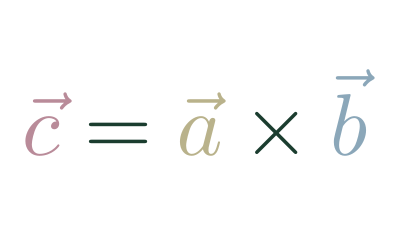An operation between two 3d vectors that returns a vector.

• ## Matrix Determinate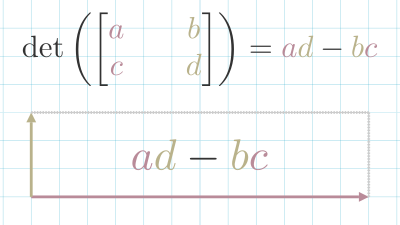A measure of how a matrix scales space.

• ## Basis Vectors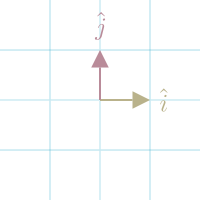The set of vectors that defines space.

• ## Roblox CFrame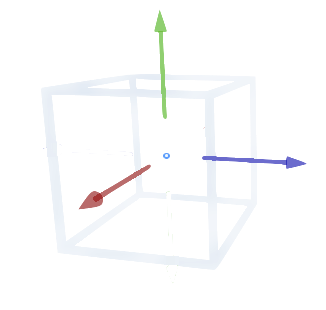In Roblox, a CFrame (coordinate frame) is an object that encodes position and rotation in 3D space.

• ## Identity Matrix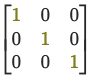When you multiply a matrix $(A)$ by the Identity Matrix $(I)$, you get the original matrix back.

$A …$

• ## Law Of Cosines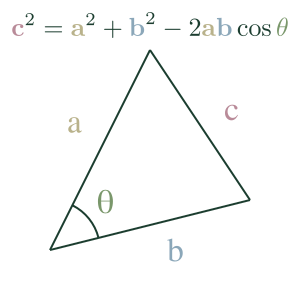The Law of Cosines expresses the relationship between the length of a triangle's sides and one of its angles.

• ## Roblox Vector3

In Roblox, a Vector3 defines a Vector with three components. We use it for setting the position, rotation, and size of elements.

For example, a …

• ## Presizing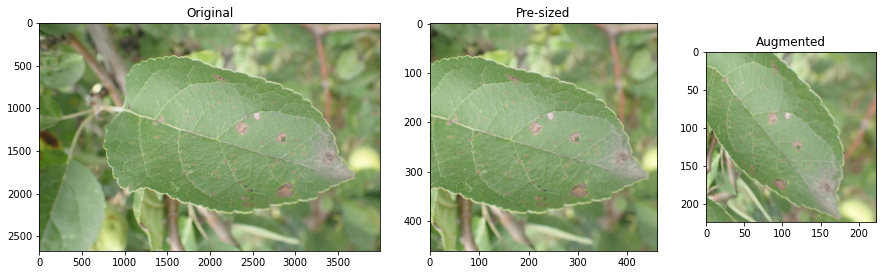Pre-sizing is a technique for preparing images for training a neural network.

In standard image pipelines, images are resized to a size suitable for our …

• ## Cross-Entropy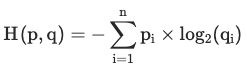Cross-entropy measures the average number of bits required to identify an event if you had a coding scheme optimised for one probability distribution $q …$

• ## Domain ShiftWhen production data diverges significantly from the training dataset